Trial ends in
JoVE Science Education
Structural Engineering

A subscription to JoVE is required to view this content.
You will only be able to see the first 20 seconds.

Buckling of Steel Columns

# Buckling of Steel Columns

### Overview

Source: Roberto Leon, Department of Civil and Environmental Engineering, Virginia Tech, Blacksburg, VA

In the design of civil works, it is important to deliver structures that are not only safe under unexpected loads, but also provide excellent performance under everyday loads at a reasonable economic cost. The latter is often tied to minimum use of materials, ease of fabrication, and rapid construction in the field. Structures made of steel members can be very economical because of the great strength of the material and the extensive prefabrication of their members and connections, which help maximize the speed of construction on site. Generally, the skeleton of a steel structure will be very slender as compared to a reinforced concrete one. While its behavior in tension is governed primarily by the strength of the material, steel in compression is governed by another failure mode common to all materials- buckling. This behavior is easily demonstrated by pressing down on a slender wooden ruler, which under a compressive load will suddenly move sideways and lose load carrying capacity. This phenomenon will occur in any slender member of a structure. In this lab, we will measure the buckling capacity of a series of slender aluminum columns to illustrate this failure mode, which over time has led to many catastrophic failures including that of the Quebec River Bridge, which was erected in 1918.

### Principles

Since the phenomenon of buckling is easily observable, it was well known since antiquity, but analytical insights into the problem of buckling did not gain attention until the 1700s when the mathematical underpinnings of physics became a popular subject of study. Leonhard Euler, a famed Swiss mathematician, was the first to provide the solution to the buckling load of a simply supported column in 1742. Euler formulated his solution by reasoning that a perfectly straight column could be in equilibrium in two configurations: an undeformed one and a deformed one (slightly bent position).

For the deformed column, Euler postulated that the equilibrium in a slightly bent configuration in which the external moments, given by the load P acting at an eccentricity y, are balanced by the internal moments (M):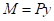(Eq. 1)

The quantity y is the lateral displacement along the length z. The first derivative of y is the slope, and the second derivative of y is the curvature of the member. The internal resistance is proportional to the curvature, or to the internal moment divided by the bending stiffness (EI), so that: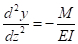(Eq. 2)

In this equation E is the modulus of elasticity and I is the moment of inertia, a geometrical property of the section. Substituting (Eq. 2) into (Eq. 1) and setting it equal to zero gives the traditional differential equation of buckling, where y is the horizontal deformation, and k is a substitution variable used to simplify the equations.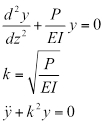(Eq. 3)

If we assume that the column deformation along its length z is given by:(Eq. 4)

and that the column has pinned ends, and that these ends do not displace laterally with respect to one another, then the boundary condition at z = 0 and L, the lateral displacement, is zero. Thus,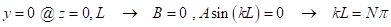(Eq. 5)

where N= 1,2, …. The lowest value for N is 1, which is the elastic buckling load (P critical or P cr). For a column with pinned ends, (i.e. with ends free to rotate, but not translate as the given boundary conditions above) Pcr is given by the Euler buckling load:(Eq. 6)

It is important to note that this equation does not contain any terms related to the strength of the material, only to its modulus of elasticity (E), dimensions, and length. The moment of inertia (I) of a section made up of rectangular parts is given by the summation about the centroid of the section of two components: the moment of inertia of the individual rectangle (bd3/12) plus its area (A) times its distance from the centroid of the entire section (d):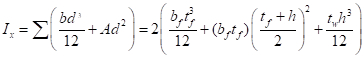(Eq. 7)

Eq. 7 highlights that the value of I can be increased significantly by putting most of the material as far away from the centroid as possible (i.e. by maximizing d). For example, for a fixed total area of 13 in.2, one could opt for two distributions: (a) one single rectangle of 13 in. x 1 in., resulting in a total I of 183 in.4, or (b) a W-shaped section with two flanges of 6.5 in. x 0.45 in. connected with a web of 0.35 in. x 19.1 in., resulting in a total I of 761 in.4. Clearly the W-shape will be a much more efficient use of the material with respect to compression, as it will provide over 4 times greater buckling capacity. The actual standard AISC W-shape with an area of 13 in.2, a W21x44 (nominal depth of 21 in. and a weight of 44 lbs. per foot) provides an I of 843 in.4 or over 4.5 times that of the rectangular section.

The relationship between the moment of inertia (I) and area (A) is defined by the radius of gyration (r):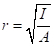(Eq. 8)

The buckling capacity is sometimes expressed as a critical stress (Fcr) by dividing the critical load by the area: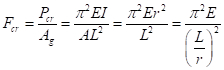(Eq. 9)

One needs to keep in mind that there are some limitations inherent in the derivation of Eq. (6) and Eq. (9) in that they assume:

1. Purely elastic behavior, and thus they are valid only up to the proportional limit of the material.
2. The load is applied at the centroid of the column, which is hard to achieve in practice. Thus, accidental initial eccentricities will play a role in design.
3. The column is initially perfectly straight. Since steel shapes are produced by a rolling process, they will have a camber and sweep (i.e., they will be slightly curved along both principal axes). These initial imperfections are small, on the order of L/1000, but will make real column behavior deviate from that of an idealized column.
4. A deflected shape, which in our case took the form of a trigonometric function (i.e., a combination of sine and cosine functions). For this case, we actually used the correct analytical solution, but that is not always possible. In general, any function approximating the correct solution will give a satisfactory approximate, but not an exact solution.
5. Idealized end conditions. In order to solve for the buckling load, the boundary conditions to the mathematical problem have to be established, and we assumed that the column had pinned ends. In addition, it was assumed that the ends of the column did not translate laterally with respect to one another (i.e., this is the sway-prevented case, which occurs in braced frames, as opposed to the sway-permitted case, which occurs in unbraced frames). In real life, these idealized conditions can only be approximated.
6. The absence of any residual stresses, which arise from the cooling and rolling of the steel shapes during production. These stresses results in yielding earlier than expected and the loss of moment of inertia, as the sections yielding have a modulus of elasticity of zero. As the column stiffness decreases, the capacity of the column has to decrease, since Eq. 1 has EI in the numerator.

The second, third, and last limitations are generally treated together as initial imperfections, and their magnitudes are keyed to established construction and fabrication tolerance. Column design curves have been developed that address these issues satisfactorily.

A structural/mechanical system is said to be imperfection sensitive if the load carrying capacity of the imperfect system is substantially less than that of the perfect system. Conversely, a system is said to be imperfection insensitive if there is no loss of loadcarrying capacity because of the imperfections. A column is said to be a perfect column if it is straight, and the load is concentric. While this is impossible in practice, we are fortunate because columns are imperfection insensitive, and thus will not have any sudden loss of load carrying capacity under normal loads. On the other hand, spheres and cylinders are imperfection sensitive, and as a result, much care must be given during construction of shells (domes, cooling towers, and storage tanks) and other such structures to obtain the correct geometry. The effect of the imperfections is to accelerate the rate of lateral deflection, since they tend to increase the bending moments in the column.

The limitations related to the fifth assumption, that of the boundary conditions, can be treated simply by the use of the concept of an effective length (kL). The effective length factor gives the proportion of the length between points of inflection (i.e., points of zero moment or zero curvature along the column). Therefore, Eq. (9) can be rewritten as: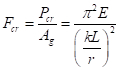(Eq. 10)

The denominator (kL/r) is known as the slenderness of the column. A low value (for example, kL/r < 20) is synonymous with a stocky column, which is not very susceptible to buckling, while a large value (for example, kl/r > 100) is synonymous with a slender column, which is very susceptible to buckling.

It should be noted that the critical stress (σcr) for design is capped by the yield strength of the material (σy). This constraint means that for any given steel strength, say σy = Fy = 50 ksi , there will be a slenderness below which buckling will not occur. If we equate σcr = 50 ksi in Eq. (10), the limit slenderness is kl/r < 75.6.

Another important caveat is that the formulation above indicates that buckling will occur suddenly as the axial load reaches its critical value (Pcr). Mathematically, this fact indicates that buckling is a bifurcation problem. Because of initial imperfections, accidental eccentricities, and residual stresses among other factors, there will be a transition between the elastic buckling stress and the squash load. The result of these initial imperfections is that in real life there will be a smooth transition between the elastic buckling curve and the yield limit states.

At this point, it is important to note that the instability or buckling phenomenon under discussion is only one of many that can occur. Instabilities occur at both the local and global level. Global level instability is when all elements (an element is defined as any rectangular section that makes up a shape) move together during buckling. Local buckling occurs when only one of the elements moves. Examples of global buckling are:

• Flexural buckling, which is the case discussed above.
• Torsional buckling, in which the section twists about its longitudinal centroid. Sections that have small torsional stiffness (J) are prone to this type of failure.
• Flexural-torsional or lateral-torsional buckling, which is a combination of the first two types of global buckling and is the predominant instability mode for beams.
• Shear buckling, in which the buckling occurs in the thin webs of deep girders due to the formation of a tension field in the diagonal direction.

Sections can also buckle locally. This is analogous to each section of the column buckling individually as a plate. Local buckling is governed by the width-to-thickness ratio (b/t) or slenderness ratio of the section and the plate's aspect ratio (b/a, where a is the length). The slenderness depends on whether both edges of the plate are connected to another section (stiffened case), or whether only one edge is connected (unstiffened case). The buckling capacity for a plate of width b and thickness t, analogous to Eq. (10) for a column, is given by:(Eq. 11)

The buckling coefficient K reflects the boundary conditions and the aspect ratio (length-to-width) of the plate. Values of K are widely available in structural design handbooks.

### Procedure

1. Obtain several long pieces of a 1in. by ¼ in. aluminum bar (6061 or similar), and cut them to lengths of 72, 60, 48, 36, 24, 12 and 8 in., respectively. Round both ends of the bars to a circumference of 1/8 in.
2. Measure the dimensions of the bar (length, width and thickness) to the nearest 0.02 in.
3. Machine two small block of steel (2 in. x 2 in. x 2 in.) to have a very smooth ½ in. circular penetration along one of its sides to serve as the column end support. Provide an insert on the opposite side, so that the block can be fixed to the testing machine.
4. Insert the blocks and a test specimen into the testing machine. Be sure to align the specimen as carefully as possible to eliminate eccentricities.
5. Set the testing machine on deflection control and program it to slowly apply a deformation of up to 0.2 in. and record load and axial deformation. The limit can be varied with the length, but the test should be stopped when the load has stabilized or when it has reached not more than a 20% load reduction from the maximum capacity.
6. Record the maximum load reached and fill in the results table.
7. Repeat steps 1.4 through 1.6 for all columns.

Buckling phenomenon is of critical importance in designing structures that are safe under unexpected loads and also provide excellent performance under everyday loads at a reasonable cost.

Due to the material's strength, the skeleton of a steel structure is very slender when compared to brick or reinforced concrete. The prefabrication of steel components increases the onsite construction speed and makes steel structures more economical than other building materials.

Under a load, the structural elements are subjected to tension or compression forces. Under tension, steel behavior is governed primarily by the strength of the material. Under compression, steel is subjected to buckling. This phenomenon occurs in any slender structure indifferent of material.

Buckling consists of a sudden sideway deflection of the column. A small increase in the applied load can lead to a sudden and catastrophic collapse of the structure. The collapse of the Quebec River Bridge due to the buckling of the lower cord members of the structure is an example of such catastrophic failure. This video will discuss the buckling failure mode and show how to determine the buckling capacity of slender columns.

A column under an axial compressive load will buckle, or suddenly move sideways, and lose load carrying capacity. Euler, a Swiss mathematician, was the first to provide the solution to the buckling load by reasoning that a perfectly straight column could be an equilibrium in two configurations: an undeformed one and a deformed one.

Euler postulated that at the equilibrium in a slightly deformed configuration, the internal moments M are balanced by the external moments given by the load P acting at an eccentricity y. The second derivative of the lateral displacement y is the curvature of the member. This quantity is proportional with the internal resistance or to the internal moment divided by the bending stiffness.

In this equation, E is the modulus of elasticity, and I is the moment of inertia, a geometrical property of the section. By substituting the first equation into the second equation, we get the differential equation of buckling, where k is a substitution variable.

Let's assume that the column deformation is given by the following function. We also assume that the column has pinned ends that do not displace laterally with respect to one another. Then, the boundary condition at Z equals zero and Z equals L is given by the lateral displacement y equals zero. As a consequence, kL equals N pi. Here, N is an integer, and its lowest value is one which is the elastic buckling load P critical. For a column with pinned ends, P critical is given by the Euler buckling load.

The critical load is the minimum load that may cause the column to buckle. Note that this equation does not contain any terms related to the strength of the material, only to its stiffness and dimensions. In order to increase the value of the critical load for a column, we can maximize the moment of inertia.

Let's consider a W-shaped section. Its moment of inertia with respect to the centroid of the section is given by the summation of the moment of inertia for each rectangle. For each rectangle, the total moment has two components. The moment of inertia of the individual rectangle, plus its area, times its distance to the centroid of the entire section. In consequence, the value of I can be increased significantly by putting most of the material as far away from the centroid as possible.

The relationship between the moment of inertia I and area A is defined by the radius of gyration r. The buckling capacity is sometimes expressed as a critical stress, Fcr, by dividing the critical load by the area. Keep in mind that there are some limitations inherent in the derivation of buckling capacity with Euler theory, since we assume: purely elastic behavior, load applied at the centroid of the column, the column is initially perfectly straight, a deflected shape which gives an exact solution, idealized boundary conditions, the absence of any residual stresses.

These limitations are generally treated as imperfections, and their magnitudes are key to established construction tolerance. The limitations related to the boundary conditions can be treated by introducing in the expression of Euler buckling capacity an effective length factor, k. The denominator is known as the slenderness of the column. A low value of this factor, for example less than 20, is synonymous with a stocky column. While a large value, for example higher than 100, is synonymous with a slender column very susceptible to buckling.

Let's plot now the critical stress as a function of the effective slenderness lambda. The critical stress is capped by the yield strength of the material. Meaning that for any given steel strength, there will be a value of the slenderness below which buckling will not occur. Euler formulation indicates that as the axial load reaches its critical value, buckling will occur suddenly. However, because of structural imperfections, there is a transition between the elastic buckling stress and the squash load. As a result, in real life there will be a smooth transition between the elastic buckling curve and the yield limit states.

Now that you understand Euler Buckling Theory, let's use this to analyze the buckling capacity of slender metal columns.

Have a set of testing specimens manufactured from one inch by a quarter inch aluminum bar cut to lengths ranging from eight inches to 72 inches. Machine both ends of each specimen to a radius of 1/8 of an inch. Measure the dimensions, length, width, and thickness, of each specimen to the nearest 0.02 inches.

Manufacture a testing fixture for the specimens from two small blocks of steel approximately two inches on a side. Machine a very smooth, half-inch circular groove along one side to mate with the specimens. On the sides opposite the groove, an insert should be provided for fixing to the universal testing machine. Before you begin testing, familiarize yourself with the machine and all safety procedures. Insert the steel blocks into the testing machine with a specimen and ensure that everything is carefully aligned to eliminate eccentricities.

In the test software, set the machine to deflection control and have both load and axial deformations recorded. Program the machine to slowly apply to deformation of up to 0.2 inches and then begin the test. This limit can be varied with specimen length, but the test should be stopped when the load has stabilized or before it drops more than 20% from the maximum capacity.

When the test is complete, record the maximum load reached for this specimen. Then reset the machine and repeat the testing procedure for the remaining specimens. After all of the specimens have been tested, you are ready to look at the results.

First, calculate the slenderness parameter lambda, and then using Euler's formula, calculate the buckling stress for each specimen. Next, use the material strength to calculate the characteristic slenderness below which buckling will not occur.

Plot the ratio between the buckling stress and the material strength as a function of the slenderness ratio. On the same graph, also plot for all specimens the measured buckling load normalized with the material strength. Now compare the measured values with the calculated values.

The experimental results show two distinct regions. When the columns are relatively long, the data follow the Euler buckling curve. As the columns begin to get shorter, the critical load begins to approach the strength of the material. At this point, the behavior shifts from a purely elastic one to a partial inelastic one that approaches asymptotically the squash load of the column.

The importance of buckling is well-recognized in the construction industry where the design of steel structures is predicated on a good grasp of buckling issues.

Economy and design requires that the volume of material be minimized while also preventing buckling instabilities. In bridge structures, this is achieved by the widespread use of W-shaped members, and by adding stiffeners in the bridge plate girders to reduce the buckling lengths in plates.

A structural system is said to be imperfection sensitive if its load carrying capacity is substantially less than that of the perfect system. While columns are imperfection insensitive, spheres and cylinders are sensitive to imperfections and, as a result, much care must be given during construction of shells; for example, domes, cooling towers, and storage tanks, and other such structures to obtain the correct geometry.

You have just watched JoVE's introduction to buckling of steel columns. You should now understand how to apply Euler's Theory of Buckling to determine the buckling capacity of slender metal members.

Thanks for watching!

### Results

Plot the results from the table as buckling stresses vs. slenderness (kL/r), along with the curve given by Eq. 9. Compare your results with the predicted values. The experimental results shows two distinct regions. When the columns are relatively long, the critical load is given by multiplying Eq. 9 by the area of the column. As the columns begin to get shorter, the critical load begins to approach the strength of the material. At this point the behavior shifts from a purely elastic one to a partial inelastic one that approaches asymptotically the squash load of the column. When a column buckles elastically, deformation can become every large suddenly and trigger failures either in the buckled member or in adjacent ones that become overloaded as the buckled member sheds its loads. Thus, in design it is important to prevent elastic buckling failures in primary structural members.

### Applications and SummaryX